An inverting op amp is an operational amplifier circuit with an output voltage that changes in the opposite direction as the input voltage. The operational amplifier inverting input receives feedback from the output of the amplifier. Here will tells you how to design it and its circuit exa

Introduction
The operational amplifier has a non-inverting input terminal and an inverting input terminal in the electronic circuit. The polarity of the input terminal and the output terminal are the same is a non-inverting amplifier, and the polarity of the input terminal and the output terminal are opposite called inverting amplifier. The inverting amplifier circuit has the function of amplifying the input signal as inverting output.

1 Inverting Amplifier Basics Overview
1.1 Working Principle
As shown in the figure, the inverting amplifier circuit has the function of amplifying the input signal as inverting output. "Inverting" means that the positive and negative signs are reversed. This amplifier uses negative feedback technology, which used to return a part of the output signal to the input. In Figure, the wiring method of connecting the output Vout to the inverting input terminal (-) via R2 is negative feedback.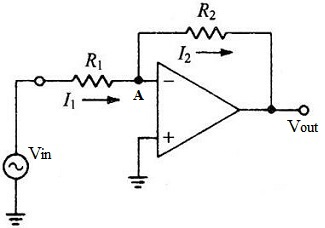Fig 1. Inverting Amplifier Circuit

Operational amplifiers have such characteristics. When the power supply voltage is not applied to the output terminal, the non-inverting input terminal (+) and the inverting input terminal (-) are considered to have the same voltage, that is to say, it can be regarded as a virtual short circuit. Therefore, when the positive input terminal (+) is 0V, the voltage at output is also 0V.
The input impedance of the operational amplifier is extremely high, and there is basically no current in the inverting input terminal (-). Therefore, when the current flows to R2, the I1 and I2 are basically equal. Based on the above conditions, using Ohm's law for R2, we get Vout=-I1xR2. I1 is negative because I2 flows from point A where the voltage is 0V. From another point of view, when the input voltage of the inverting input terminal (-) rises, the output will be inverted and amplified greatly in the negative direction. Since the output voltage in the negative direction is connected to the inverting input terminal via R2, the voltage rise of the inverting input terminal (-) will be blocked. Both the inverting input terminal and the non-inverting input terminal voltage become 0V, and the output voltage is stable.

1.2 Inverting Amplifier Gain Calculator
Calculate the gain through the relationship between the input and output in this amplifier circuit. The gain of an inverting op amp is the ratio of the feedback resistance to the input resistance, that is, the ratio of Vout to Vin, and the formula is Vout/Vin= (-I1xR2) /(I1xR1)=-R2/R1. The resulting gain is negative, indicating that the waveform is inverting.
The current flowing through R1: I1=(Vi-V-)/R1...a
The current flowing through R2: I2=(V--Vout)/R2……b
V-=V+=0………………c
I1=I2……………………d
Solve the above algebraic equations to get Vout=(-R2/R1)*Vi.
This is the input and output relationship of the inverting amplifier circuit.

1.3 Inverting Amplifier Features
It can reduce the input impedance or keep a certain value.
It can be used as a current input type.
Virtual short-circuit point is generated at a certain potential.
Its positive input port is free.
If the signal source impedance is low, it is easier to obtain a required S/N.
The magnification is -Rf/R.
1.4 Inverting Amplifier Functions
The inverting amplifier is the basic gain stage in the CMOS circuit. It adopts a common source structure, and the load can be an active load or a current source.
Advantages: The potential of the two input terminals is always approximately zero (the non-inverting terminal is grounded, and the inverting terminal is virtual ground). With only differential mode signals,it has strong anti-interference ability.
Disadvantages: The input impedance is very small, equal to the resistance of the series resistance from the signal to the input.

2 Inverting Amplifier Applications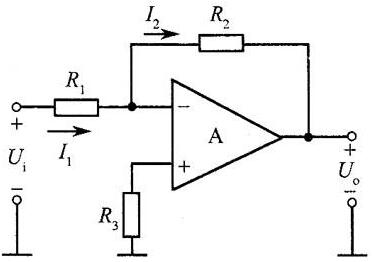Fig 2. Basic Inverting Amplifier Circuit

1) As a Integrator
The original resistor R2 of the inverting amplifier is replaced by a capacitor C2. At this time, the relationship between the input signal Vi and the output signal Vo forms an integral relationship.
2) As a Differentiator
Replace the original resistor R1 of the inverting amplifier with an electric capacitor C. At this time, the relationship between the input signal Vi and the output signal Vo is differential.
If the inverting amplifier is slightly changed, the relationship between the input signal and the output signal Vo at this time, if R1 = R2 = R3 =...= Rn = Rf, it can be simplified to Vo = -(V1+V2+V3+.. .+Vn), is additive.

3 Inverting Amplifier Explain with Diagrams: NE5532
The equivalent resistance seen between the input terminal and the ground of the inverting amplifier circuit is equal to the equivalent resistance between the input terminal and the virtual ground, so the input resistance of the circuit is Ri=R. It can be seen that although the input resistance of the ideal operational amplifier is infinite, the input resistance of the inverting proportional arithmetic circuit is not large because of the parallel negative feedback introduced by the circuit.Fig 3. NE5532 Audio Amplfier Circuit

In order to increase the input resistance, R must be increased. For example, when the scale factor is -50, if Ri=10kΩ, R should be 10kΩ and Rf should be 500kΩ. If Ri=100kΩ, R should be 100kΩ and Rf should be 5MΩ. In fact, when the resistance in the circuit is too large, on the one hand, due to the process, the stability of the resistance is poor and the noise is large, on the other hand, when the resistance is of the same order of magnitude as the input resistance of the integrated op amp, the proportional coefficient -Rf/R of the circuit will change greatly, and its value will not only be determined by the feedback network. Therefore, it is necessary for practical applications to use a resistor with a smaller resistance value to get a larger scale factor and a larger input resistance.
Look at the following two simulations: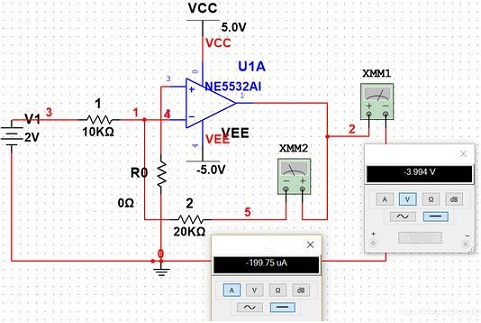Fig 4. Current and Voltage Measuring

From above, it doesn't matter without R0, take a look at this below.Fig 5. Current and Voltage Measuring

Their output is different, if the expected result is -4V. The reading value -3.608V obviously does not meet the design requirements. We now know that the resistance value of Rs in Figure is orders of magnitude greater than the input resistance of the integrated operational amplifier. Next, if have R0, look at the picture below.Fig 6. Current and Voltage Measuring

It can be seen from Figure 4 that after adding R0, the output of the op amp is normal. Why is this?
In a TTL circuit, the transistor has a bias current, which will produce a DC voltage drop on the feedback resistor of the e pole. Although it is small, the amplifier has a high amplification factor, which affects the output accuracy greatly. A resistor in the same value as the negative terminal is connected to the positive terminal to cancel the effect of the bias current.
R0 is a compensation resistor, which minimizes the bias current error to ensure the symmetry of the differential amplifier circuit of the input stage. When its value is u=0 (that is, the input terminal is grounded), the inverting input terminal is always the equivalent resistance, that is, the parallel connection of the resistance of each branch, so R0=Rs/Rf, through Rf in the circuit, induce negative feedback. According to practical experience, it is best to add a compensation resistor to improve the stability of the circuit. Of course, it can be omitted under normal circumstances.

4 Inverting Amplifier Circuit Design
Inverting amplifiers are several commonly used amplifier types. How to use operational amplifiers design inverting amplifier circuit? The following sharing is the detailed steps.
Step 1: Determine the magnification.
As shown in the figure below, the amplification factor of the inverting amplifier is (-R2/R1).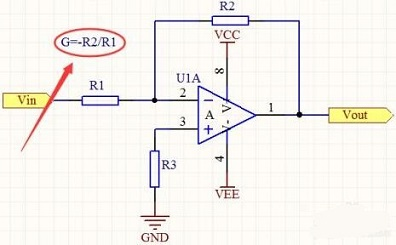Fig 7. Determine the Magnification

Step 2: Determine the supply voltage.
Ensure that the output voltage after the input voltage Vin is multiplied by the amplification factor (-R2/R1) will not exceed the power supply voltage. If it is not the rail-to-rail operational amplifier used, it is better to have a margin of 1~2V.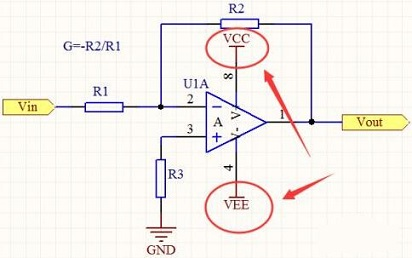Fig 8. Determine the Supply Voltage

Step 3: Determine the gain bandwidth product (GBW).
If you are amplifying an AC signal, you need to consider the gain bandwidth product. The calculation formula is: bandwidth of input signal × design gain, select an operational amplifier whose GBW is greater than the required one.Step 4: Check the bandwidth with the slew rate (SR).
If the amplifying is a large AC signal, there may be insufficient bandwidth, and it may not be accurate enough to calculate based on the gain-bandwidth product.
SR = 2*pi*f*Vp - f = SR/(2*pi*Vp), where Vp: peak output voltage.
If the calculated value f is less than the input voltage frequency, the operational amplifier is not suitable and needs to be replaced. The actual bandwidth should be the smaller of the gain bandwidth product and the slew rate.Step 5: Determine the input offset voltage.
Since the offset voltage is also a DC signal, if it is to amplify the DC signal, it should be noted that the offset voltage will also be amplified by the corresponding multiple. If the accuracy is high, try to choose an operational amplifier with a small offset voltage.Step 6: Determine the resistance value.
In step 1, the ratio of R1 and R2 has been determined. R1 is generally 1k~10k. A small value is prone to cause gain errors, and a larger value will increase noise (resistor thermal noise). R2 takes the resistance value corresponding to the multiple of R1.
It is better to choose the chip resistor, because the parasitic parameters are small.
R3=R1/R2, if R1 and R2 are connected in parallel, which are not required.